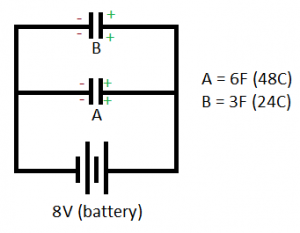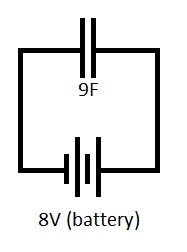# Capacitors in Parallel

• Voltage remains the same
• Equivalent Capacitance = C1 + C2 + C3 ….• C = Q / V
• For A:
• 3 = Q / 8
• Q = 24
• For B:
• 6 = Q / 8
• Q = 48
• So C1 (24) + C2 (48) = 72C (equiv)
• Ceq = 6F + 3F = 9F
• Capacitor A has twice the Farad (F) –> therefore twice the charge

The above is the equivalent to:• C = Q / V
• 9F = Q / 8V
• Q = 72C